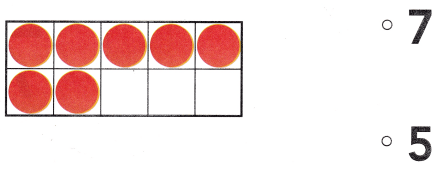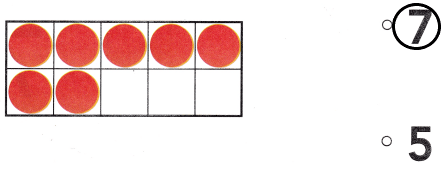# Texas Go Math Kindergarten Lesson 4.3 Answer Key Model and Count 7

Refer to our Texas Go Math Kindergarten Answer Key Pdf to score good marks in the exams. Test yourself by practicing the problems from Texas Go Math Kindergarten Lesson 4.3 Answer Key Model and Count 7.

## Texas Go Math Kindergarten Lesson 4.3 Answer Key Model and Count 7

Explore

DIRECTIONS: Model 6 objects. Show one more object. How many are there now? Tell a friend how you know. Draw the objects.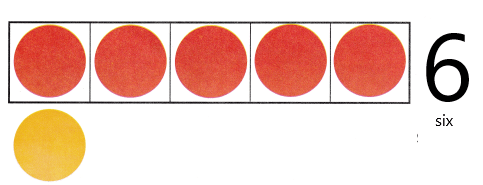Share and Show

DIRECTIONS: 1. Place counters as shown. Count and tell how many counters. 2. 5 and how many more are 7? Write the number. 3. Place counters in the ten frame to model seven. Draw the counters. Tell a friend what you know about the number 7.

Question 1.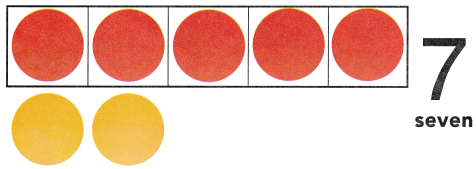There are 7 counters in the above ten-frame.

Question 2.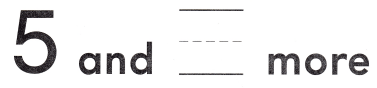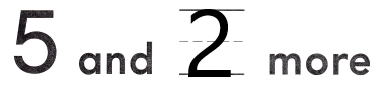Explanation:
7 is 5 and 2 more.So, i wrote the number 2.

Question 3.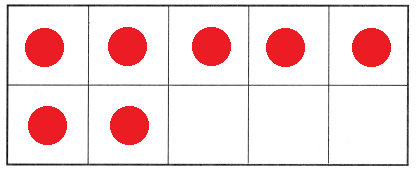Explanation:
I drew 7 counters in the above ten-frame.There are 7 counters.

DIRECTIONS: 4. Place two-color counters in the ten frame to model the different ways to make 7. Write to show some pairs of numbers that make 7.

Question 4.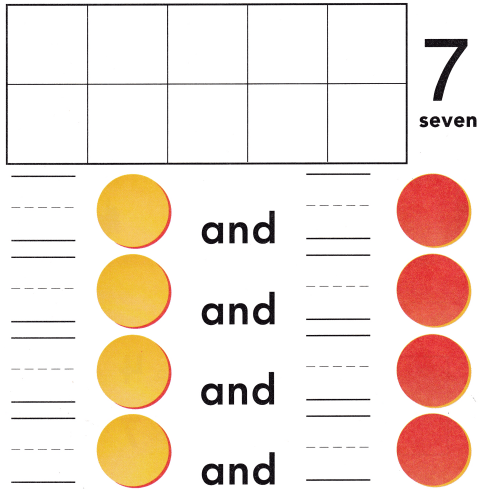Explanation:
I placed two-color counters 6 red and 1 yellow counters in the ten frame to model the different ways to make 7 and i wrote to show some pairs of numbers that make 7, they are 6 yellow and 1 red counter, 5 and 2, 4 and 3 and 3 and 4.

HOME ACTIVITY • Ask your child to show a set of six objects. Have him or her show one more object and tell how many objects are in the set.

DIRECTIONS: 5. A carousel needs seven horses. Count the horses in each set. Circle the sets with seven horses. 6. Choose the correct answer. What number does the model show?

Problem Solving

Question 5.Explanation:
I circled those groups of horses that are 7 in number.

Question 6.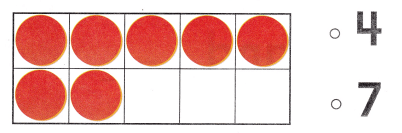Explanation:
I counted the number of counters.There are 7 counters so, i circled the number 7.

### Texas Go Math Kindergarten Lesson 4.3 Homework and Practice Answer Key

DIRECTIONS: 1. Draw some red and yellow counters to make 7. Write to show the number of red and number of yellow counters. 2. Draw some red and yellow counters to make 7 another way. Write to show the number of red and number of yellow counters.

Question 1.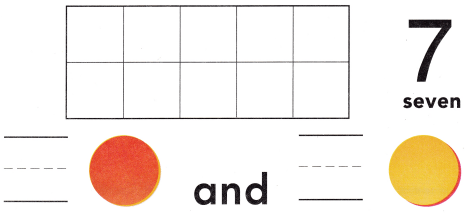Explanation:
I drew 5 red and 2 yellow counters to make 7 and wrote 5 and 2 to show the number of red and number of yellow counters.

Question 2.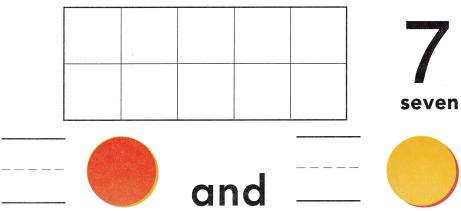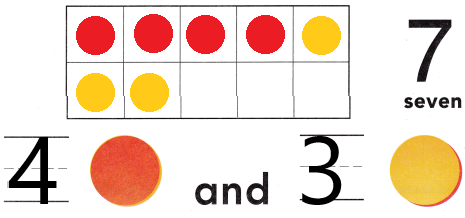Explanation:
I drew 4 red and 3 yellow counters to make 7 and wrote 4 and 3 to show the number of red and number of yellow counters.

DIRECTIONS: Choose the correct answer. 3-4. What number does the model show?

Lesson Check

Question 3.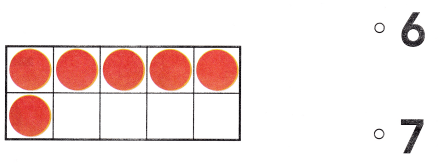Explanation:
I counted the number of counters.There are 6 counters.So, i circled the number 6.

Question 4.